# Go Math Grade 4 Answer Key Homework FL Chapter 11 Angles Review/Test

Go Math Grade 4 Answer Key Homework FL Chapter 11 Angles Review/Test helps to test your knowledge on this topic. Learn the methods to measure the angles on our Go Math Grade 4 Answer Key. Get step by step explanation with diagrams on Go Math Grade 4 Answer Key Homework FL Chapter 11 Angles Review/Test.

## Go Math Grade 4 Answer Key Homework FL Chapter 11 Angles Review/Test

### Review/Test – Page No. 439

Choose the best term from the box.Question 1.
The size of an angle can be measured using a tool called a
______________ .
________

The size of an angle can be measured using a tool called a Protractor

Question 2.
___________ is the direction in which the hands of a clock move.
________

Clockwise is the direction in which the hands of a clock move.

Tell what fraction of the circle the shaded angle represents.

Question 3.$$\frac{□}{□}$$

Answer: $$\frac{1}{4}$$

Explanation:
The figure shows that the 1/4th part of the circle is shaded. So, the fraction of the shaded angle is 1/4.

Question 4.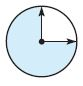$$\frac{□}{□}$$

Answer: $$\frac{3}{4}$$

Explanation:
It has completed a 3/4 turn. So, the fraction of the shaded part is 3/4.

Question 5.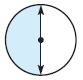$$\frac{□}{□}$$

Answer: $$\frac{1}{2}$$

Explanation:
From the figure, we can see that the circle is rotating in the anti-clockwise direction. And it has completed the half turn.
Thus the fraction is 12 turn counterclockwise

Use a protractor to draw the angle.

Question 6.
68°
Type below:
________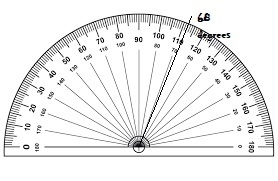Question 7.
145°
Type below:
________Question 8.
Use a protractor to find the measure of each angle. Label each angle with its measure. Write the sum of the angle measures as an equation.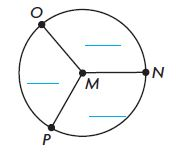Explanation:
By using the protractor we can measure each angle of the above circle.
∠NMO = 110°,
∠OMP = 120°,
∠NMP = 130°

### Review/Test – Page No. 440

Question 9.
Which describes the turn the angle on the circle shows?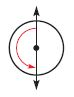Options:
a. 90° clockwise
b. 90° counterclockwise
c. 180° clockwise
d. 180° counterclockwise

Explanation:
By seeing the above circle we can say that it turns counterclockwise at 180°.
Thus the correct answer is option d.

Question 10.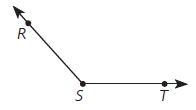Options:
a. acute; 48°
b. obtuse; 48°
c. obtuse; 132°
d. obtuse; 148°

Explanation:
By using the protractor we can measure the angle of the above figure.
The above figure is greater than 90 degrees, so it is an obtuse angle. The measure of the angle is 148 degrees.
Thus the correct answer is option d.

Question 11.
The pocket watch was invented in 1524. The time is 6 P.M. After 1 hour, how many degrees does the minute hand turn?Options:
a. 45°
b. 90°
c. 180°
d. 360°

Explanation:
Pocket watches consist of a circular face and three hands that complete a full revolution at different rates: the second hand takes 60 seconds, the minute hand takes 60 minutes, and the hour hand takes 12 hours.
There are 60 seconds in one hour, so in one hour the minute hand has completed a single revolution.
Circles contain 360 degrees so the minute hand, by completing one circle, has traveled 360 degrees after one hour.
Thus the correct answer is option d.

### Review/Test – Page No. 441

Question 12.
What is the unknown angle measure?Options:
a. 25°
b. 115°
c. 125°
d. 180°

Explanation:
Sum of the angles = 180°
65° + x° = 180°
x° = 180° – 65°
x° = 125°
Thus the correct answer is option c.

Question 13.
Which equation can you use to find the ∠WRT?Options:
a. 84° + 69° = ■
b. 84°− 69° = ■
c. 84° × 69° = ■
d. 84° − 153° = ■

Answer: 84° + 69° = ■

Explanation:
To find the unknown angle, we have to do the sum of two angles.
84° + 69° = ■
Thus the correct answer is option a.

Question 14.
If an angle measures 100º, through what fraction of a circle does the angle turn?
Options:
a. $$\frac{1}{100}$$
b. $$\frac{1}{4}$$
c. $$\frac{100}{360}$$
d. $$\frac{1}{2}$$

Answer: $$\frac{100}{360}$$

Explanation:
The complete angle is 360°
The angle measures 100º
= $$\frac{100}{360}$$
Thus the correct answer is option c.

### Review/Test – Page No. 442

Question 15.
How many right angles are there in an angle that turns through 360º? Explain how you know.
______ right angles

Explanation:
A circle has 4 right angles. So, an angle that turns through 360º has four right angles.

Question 16.
Soccer practice began at 2:30 P.M. and stopped at 3:00 P.M. because of rain. During this time, through what fraction of a circle did the minute hand turn? How many degrees did the minute hand turn? Explain.

Explanation:
A minute watch covers 360 degrees every 60 minutes. In 30 minutes the watch, therefore, covers 180 degrees.

Question 17.
Charlotte divided a whole pizza into 4 pieces. One piece formed a straight angle. One piece formed a right angle. Two pieces formed acute angles with the same degree measure.
A. Draw angles to represent the 4 pieces.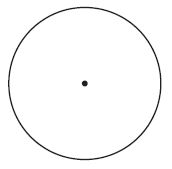Question 17.
B. Label each angle with its degree measure.Question 17.
C. Label each angle as a fraction of a circle.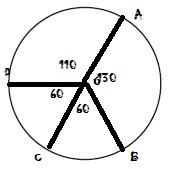Question 17.
D. Write an equation that represents the degree measure of the whole pizza as the sum of the measures of its parts.